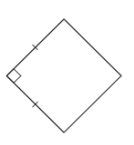Identify the Shape
True or False
Find
Sometimes, Always or Never
Wild Card
100

Which quadrilaterals always have diagonals that are congruent?

Rectangles and squares

100

False

100

Find m<PQR in the Rhombus.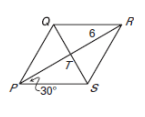m<PQR=120

100

A square is a rectangle.

Always

100

How many types of special parallelograms did we learn about?

3

200

Which quadrilaterals always have diagonals that are perpendicular?

Rhombuses and squares

200

A parallelogram with 4 right angle is always a rectangle.

True

200x = 1

y = 1/2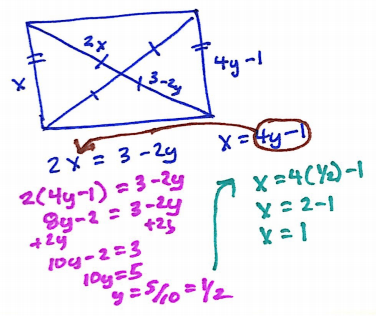200

A rectangle is a square.

Sometimes

200

What were 2 properties we used to prove parallelograms based on their sides?

Parallel & congruent

300

Which quadrilaterals have diagonals that DO NOT bisect opposite angles?

Rectangles and parallelograms

300

All rhombuses are squares

False

300

Find the value of z & w in the parallelogram.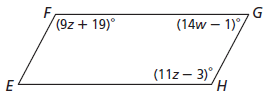z = 11, w = 4.5

9z+19=11z-3, 19=2z-3, 22=2z, z=11

plug in 11 ... m<H= 11(11)-3 = 118

m<G + m<H = 180, so m<G=62

solve ... 14w-1=62, w=4.5

300

A rectangle has perpendicular diagonals.

Sometimes

300

How many properties are shared between all the special parallelograms? Then name them.

5:

Opposite sides are parallel

Opposite sides are congruent

Opposite angles are congruent

Consecutive angles are supplementary

Diagonals bisect each other

400

Which quadrilaterals always have diagonals that bisect each other?

Parallelograms, rectangles, rhombuses, and squares

400

A parallelogram with perpendicular diagonals is a rhombus.

True

400

Find WZ if VZ= 6 and WX = 10WZ = 8

6+ (WZ)2 = 102

400

A parallelogram has diagonals that bisect each other.

Always

400Diagonals bisect each other making it at least a parallelogram, but they are also perpendicular meaning it must be a rhombus.

500

Which quadrilaterals always have consecutive angles that are supplementary?

Parallelograms, rectangles, rhombuses, and squares

500

A parallelogram with four congruent sides is a square.

False

500

If the figure is a square find the measure of each angle.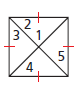m<1=90   m<2=m<3=m<4=m<5 = 45

Since diagonals are congruent, perpendicular, and bisect each other the individual triangles are all right isosceles triangle. Also the corners are 90 degrees and the diagonals bisect those angles.

The angle measures in each are 45, 45, & 90 degrees.

500

The diagonals of a rhombus are perpendicular.

Always

500